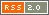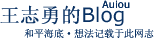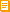订阅本博客如何订阅博客？王志勇 发表于 2019年06月30日 11:41

PHP 7引入了强类型，在报错方面比PHP 5严格了很多。所以，很多PHP 5下开发的程序，到了PHP 7会有各种报错、无法运行。其实，只要稍加改动，原来的PHP 5程序就可以兼容PHP 7的语法，但无疑改动的工作量是难以估计的，需要对每个模块都重新测试。这种改动不像前端设计所见所得，程序由于模块数量庞大，可能会有少量改动不彻底而引发bug

PHP里的单引号的执行效率更高。使用双引号，解释器需要搜索字符串里可能存在的变量，例如：\$a1="abc\$a2";，如果改成这样，解释器则不搜索(可能存在的变量)：\$a1='abc'.\$a2;

PHP 5和PHP 7在开发中，最常遇到的兼容性写法：

1. \$_POST、\$_GET、\$_COOKIE，到了PHP 7，必须给它们加上一个@号，可以防止报错，例如：

\$a1=\$_POST['c1'];
\$a2=\$_GET['c2'];
\$a3=\$_COOKIE['c3'];

\$a1=@\$_POST['c1'];
\$a2=@\$_GET['c2'];
\$a3=@\$_COOKIE['c3'];

2. 事先清空数组，防止复杂的程序里出现的数据错乱，在PHP 5里，过去我一直用这样的方法，不会报错，这是十分常用的基本语句：
\$a1='';
\$a1='abc';

\$a1=array();
\$a1='abc';

3. if语句当中出现的符合条件才赋值的变量\$a1，必须先声明这个变量\$a1。如果不事先声明，在PHP 5下永远不会报错，但是PHP 7会报错。

if (\$b1==3) \$a1='abc';

PHP 7必须这样写：
\$a1='';
if (\$b1==3) \$a1='abc';

\$a1='';
if (@\$b1==3) \$a1='abc';

2019-08-05 22:31更新

\$a1='';
if (!@\$b1) \$b1=0;
if (\$b1==3) \$a1='abc';

if (!@\$b1)的语法说明，if (!\$b1)是PHP 5的写法，它的含义相当于if (\$b1=='')，或者if (\$b1==0)，或者if (\$b1==false)。到了PHP 7，需要在\$b1前加个@以防止报错，就是if (!@\$b1)，这个写法在PHP 5下也正常运行。
(更新完毕)

4. PHP 7删去了少部分的超全局变量\$_SERVER，例如\$_SERVER['HTTP_X_FORWARDED_FOR']。这个变量的作用是获得用户的真实IP，如果使用了此变量，会报错。只能用：\$ip=\$_SERVER['REMOTE_ADDR'];

5. 在PHP 7下开发程序时，还需要尽量兼顾PHP 5的写法，使这2个版本的写法整体一致，达到互相兼容。比如PHP 7的新的写法，太空船写法：
echo \$a <=> \$b;

Feedval是PHP 5下开发，正在全力改为兼容PHP 5、PHP 7的写法。

2019-06-30 21:26更新：

2019-07-03 08:33更新：

\$a1=@\$_SERVER['HTTP_REFERER'];

2019-08-08 12:08更新：

\$b3=array();
……
for (\$g=0;\$g<=6;\$g++) \$b3[\$a1].="\n".\$o4[\$s+\$g]; //(第3行)

for (\$g=0;\$g<=6;\$g++) @\$b3[\$a1].="\n".\$o4[\$s+\$g];

<?@\$b3='abc';
echo \$b3;?>

<?if (!@\$b3) \$b3='';
……
\$b3='abc';
echo \$b3;?>

\$b3=array();
……
for (\$g=0;\$g<=6;\$g++){if (!@\$b3[\$a1]) \$b3[\$a1]='';\$b3[\$a1].="\n".\$o4[\$s+\$g];}

10条评论：
1   自由勇 2019-06-30 21:26

2   angel2018 2019-06-30 21:42

3   angel2018 2019-07-01 01:54

4   自由勇 2019-08-08 12:24
2019-08-05 22:31更新：

\$a1='';
if (!@\$b1) \$b1=0;
if (\$b1==3) \$a1='abc';

if (!@\$b1)的语法说明，if (!\$b1)是PHP 5的写法，它的含义相当于if (\$b1=='')，或者if (\$b1==0)，或者if (\$b1==false)。到了PHP 7，需要在\$b1前加个@以防止报错，就是if (!@\$b1)，这个写法在PHP 5下也正常运行。

5   自由勇 2019-08-08 12:25
2019-08-08 12:08更新：

\$b3=array();
……
for (\$g=0;\$g<=6;\$g++) \$b3[\$a1].="\n".\$o4[\$s+\$g]; //(第3行)

for (\$g=0;\$g<=6;\$g++) @\$b3[\$a1].="\n".\$o4[\$s+\$g];

<?@\$b3='abc';
echo \$b3;?>

<?if (!@\$b3) \$b3='';
……
\$b3='abc';
echo \$b3;?>

\$b3=array();
……
for (\$g=0;\$g<=6;\$g++){if (!@\$b3[\$a1]) \$b3[\$a1]='';\$b3[\$a1].="\n".\$o4[\$s+\$g];}

6   angel2018 2019-08-15 03:36

:)

 发表评论： 名字： (*必填) 博客： (可省)

 正文：　　记住信息？ 是 否直接发送Trackback到此文章说明：本评论系统不支持HTML代码。(您的留言需要审核，审核规则请见这里。)

Copyright © 2006-2019 auiou.com All rights reserved.